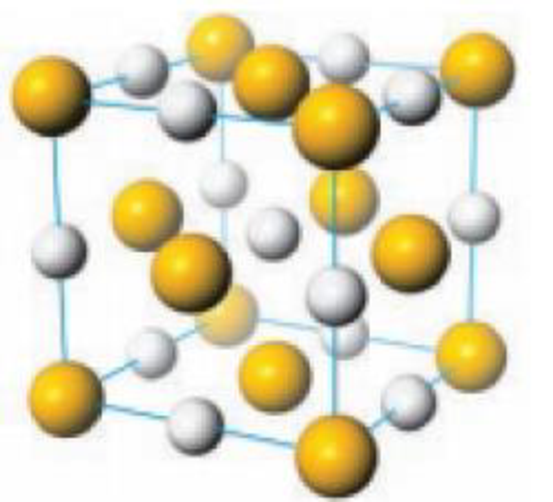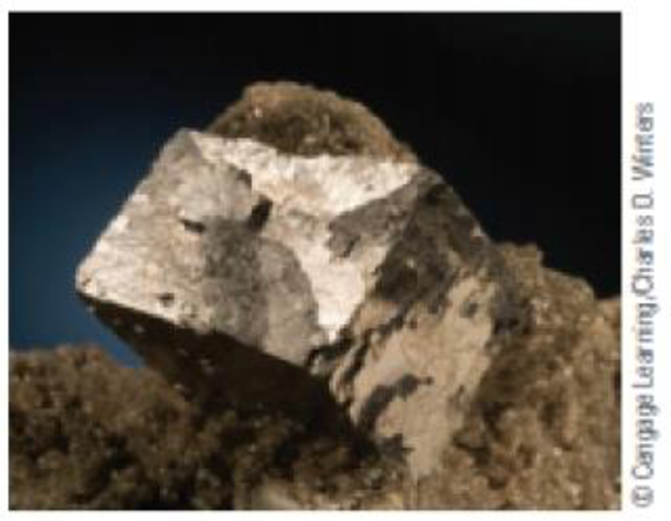Chapter 12, Problem 61IL

Chapter
Section
Textbook Problem

Like ZnS, lead(II) sulfide, PbS (commonly called galena), has a 1:1 empirical formula with a 2+ cation combined with the sulfide anion.Unit cell of PbSSample of galenaDoes PbS have the same solid structure as ZnS? If different, how are they different? How is the unit cell of PbS related to its formula?

Interpretation Introduction

Interpretation:

The structure of PbS and ZnS has to be compared and explained

Concept introduction:

The face-centered cubic system:

It has lattice points on the faces of the cube, that each gives exactly one half contributions, in addition to the corner lattice points, giving a total of 4 lattice points per unit cell

Explanation

Lead sulfide is also known as galena, Lead sulfide is form the cubic close packing (face centered cubic) arrangement, because, the ions occupy eight corners and the center of each face of the cube. Therefore it is cubic close packing arrangement; the structure of lead sulfide is similar structure with sodium chloride...

Still sussing out bartleby?

Check out a sample textbook solution.

See a sample solution

The Solution to Your Study Problems

Bartleby provides explanations to thousands of textbook problems written by our experts, many with advanced degrees!

Get Started